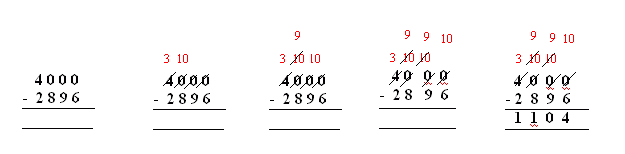Email us to get an instant 20% discount on highly effective K-12 Math & English kwizNET Programs!

#### Online Quiz (WorksheetABCD)

Questions Per Quiz = 2 4 6 8 10

### Grade 3 - Mathematics6.2 Subtracting Across Zeros

 Sometimes when you need to regroup, there is a zero in the next place. Then you need to regroup in a different way. Method: Subtract the ones, since you cannot take away from zero, you need to regroyo. The zero becomes 10. The zero in tens place becomes 9 (since the zero in ones place takes 1 from here) Again the zero in hundreds place becomes 9 (since the zero in tens place takes 1 from here) The number in thousands place becomes less by 1(since the zero in hundreds place takes 1 from here) Example:In ones place, 0 becomes 10. In tens place and hundreds place, 0 becomes 9. In thousands place, 4 becomes 3. Answer: 1104 Directions: Subtract the following. Also write at least ten examples of your own.
 Q 1: 1200 - 1123 =777687 Q 2: 1000 - 600 =500600400 Q 3: 2300 - 2126 =184174164 Q 4: 4,000 - 2896 =1,1051,2101,104 Q 5: 1600 - 1190 =110410510 Q 6: 8004 - 456 =8540754887458452 Question 7: This question is available to subscribers only! Question 8: This question is available to subscribers only!

#### Subscription to kwizNET Learning System offers the following benefits:

• Unrestricted access to grade appropriate lessons, quizzes, & printable worksheets
• Instant scoring of online quizzes
• Progress tracking and award certificates to keep your student motivated
• Unlimited practice with auto-generated 'WIZ MATH' quizzes